# Sewer Design & Design flow in Sanitary SewerTo find the design flow in sanitary sewer the following steps are followed

1. Forecast the design population (P) of the area;

2. Find the sewage flow per day by multiplying population with flow per day per capita of sewage. The sewage is taken as (70-80)% of average water supply

If (q) is the average per capita per day water consumption of water then

Qavg = Average sewage flow = (0.7 – 0.8) *q*P

Note: average value of 75% i.e. 0.75 can be used

3. Select a peaking factor (P.F) to find the peak sewage flow (Note. Sewers are designed for peak flow)

According to WASA (Water and Sanitary Agency)

P.F = 4 (for discharge upto 0.0283 m3/sec

P.F = 2 (for discharges ≥ 5.66 m3/sec

4. Calculate the allowance for industrial and commercial sewage at the rate of 3734 m3/km2/day

5. Calculate infiltration allowance of (5-10)% of average sewage flow as given by WASA

Qinf = (0.05 – 0.1) Qavg

6. Find the design sewage flow by adding Peak Flow industrial allowance and infiltration flow

Qdesign = Qpeak + Qind + Qinf

## Manning's Formula of Sanitary Sewer Equation

Normally, Manning’s formula is used for design of sanitary sewer, which is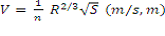Where

V = Velocity of sewage flow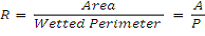S = Slope of sewer

n = roughness co-efficient (0.013 – 0.015)

n = 0.01 (for smooth inside surface like PVC etc)

n = 0.013 (for brick masonry, concrete, RCC Pipe)

n = 0.017 (for stone masonry)

n = 0.025 (for earthen channels)

## Chezy’s Formula for Sanitary Sewer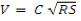Where

C= Chezy constant value of c can be obtained by using either Kutter’s formula or Bazin’s formula

## According to Kutter Sanitary Sewer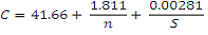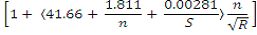## According to Bazin Sanitary Sewer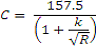Where

k = constant depending upon the nature of sewer

## Design Procedure of Sanitary Sewer

Calculate the design flow as already explained (Qd)

Select value of self cleansing velocity and compute area of pipe (sewer) by A = Q/V and diameter

Find the slope of sewer by Manning's formula

Check the velocity, it should be greater than the minimum self cleansing velocity.

AuthorsTop